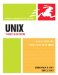# Evaluating Expressions with expr

### Evaluating Expressions with expr

Unix also provides expr, which you can use for evaluating expressions. (In this use, the term expressions refers to the mathematical, logical, scientific meaning of the word.) In addition to evaluating mathematical expressions, you can evaluate darn near anything else. The expr utility is often used in shell scriptsand you'll probably find the most value in expr in that contextbut it works just fine at the command line, too, as shown in Code Listing 15.3.

##### Code Listing 15.3. Using the expr utility, evaluating the value or the truth (or lack thereof) of expressions is straightforward.

 `[jdoe@frazz jdoe]\$ expr 3 \* 4 12 [jdoe@frazz jdoe]\$ expr 5 % 3 2 [jdoe@frazz jdoe]\$ a=\$PWD; b=\$HOME; expr\$a = \$b 1 [jdoe@frazz jdoe]\$ cd bin [jdoe@frazz bin]\$ a=\$PWD; b=\$HOME; expr\$a = \$b 0 [jdoe@frazz bin]\$ `

#### To Evaluate with expr:

• expr 3 \* 4

At the shell prompt, enter expr followed by the expression it should evaluate. In this example, we're multiplying 3 times 4. (We have to use a \ to escape (protect) the * from being interpreted as a wildcard by the shell.)

• expr 5 % 3

Determine the modulo (remainder) of 5 divided by 3. The answer appears on the next line (Code Listing 15.3).

• a=\$PWD; b=\$HOME; expr \$a = \$b

This cryptic expression sets a equal to the current directory, b equal to the home directory, and compares the two. If it returns 1 (TRue), you're in your home directory. If it returns 0 (false), you're not.Tips

• Comparisons within shell scripts allow you to check to see whether something is true or not, and then act accordingly. See Chapter 10 for more information.

• The expr man page isn't particularly helpful; search the Internet to get help with expr.UNIX, Third Edition
ISBN: 0321442458
EAN: 2147483647
Year: 2006
Pages: 251

Similar book on Amazon